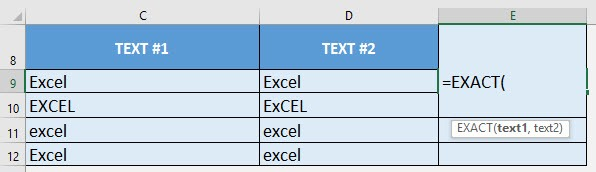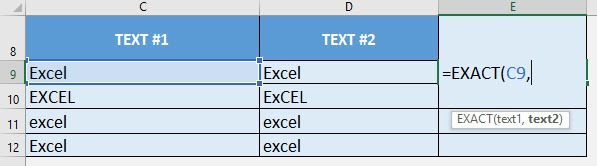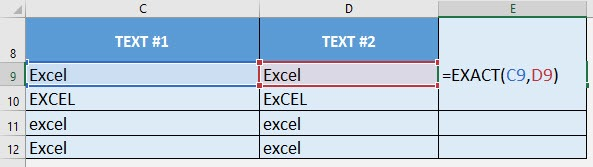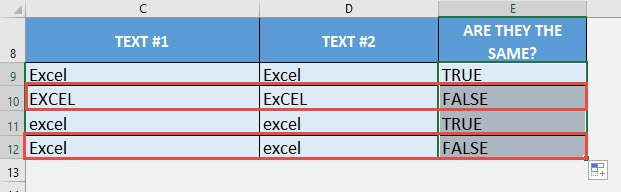What does it do?

Compares two texts in a case-sensitive manner if they are the same

Formula breakdown:

=EXACT(text1, text2)

What it means:

=EXACT(first text to be compared, second text to be compared)

We can easily compare two texts if they are equal or not with the EXACT formula.

But I can hear you say now, hold on, I can easily do that with the equal (sign) operator! The difference is, equal compares text and does not consider case sensitivity. However the EXACT formula compares text in a case-sensitive manner.

I explain how you can do this below:STEP 1: We need to enter the EXACT function in a blank cell:

## =EXACT(STEP 2: The EXACT arguments:

## text1

What is the first text to be compared?

Select the cell containing leftmost text that you want to compare:

## =EXACT(C9,## text2

What is the second text to be compared?

Select the cell containing rightmost text that you want to compare:

## =EXACT(C9, D9)Apply the same formula to the rest of the cells by dragging the lower right corner downwards.

You can see that the Excel values that have different capital letters have a FALSE result! (e.g. EXCEL vs. ExCEL)How to Use the EXACT Formula in Excel